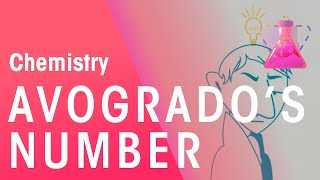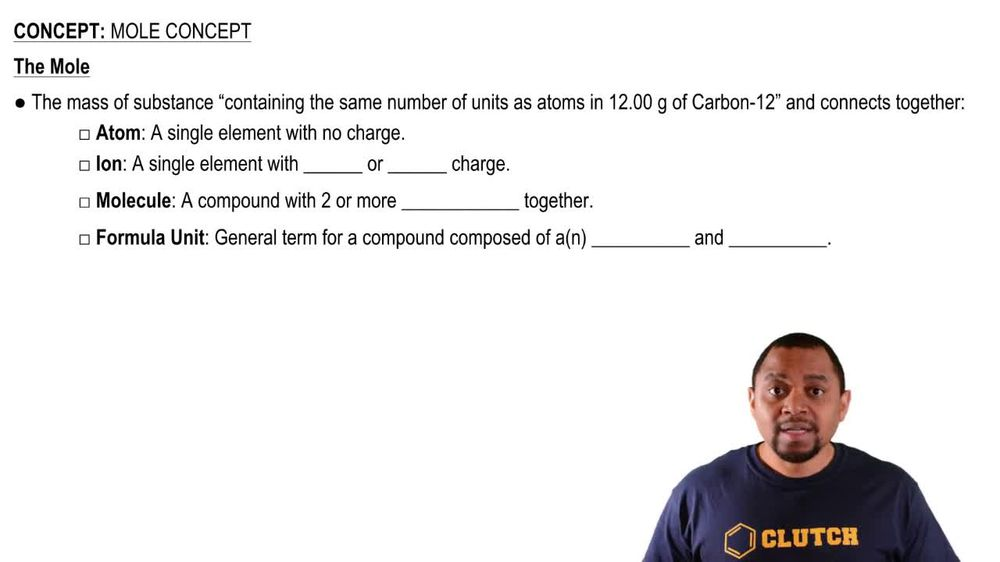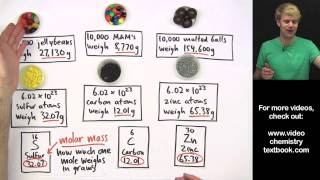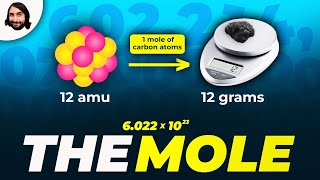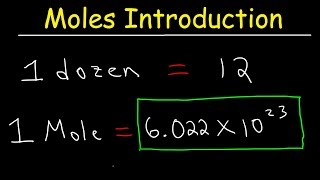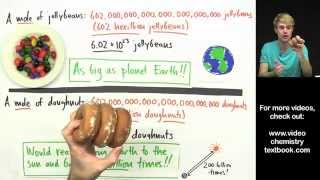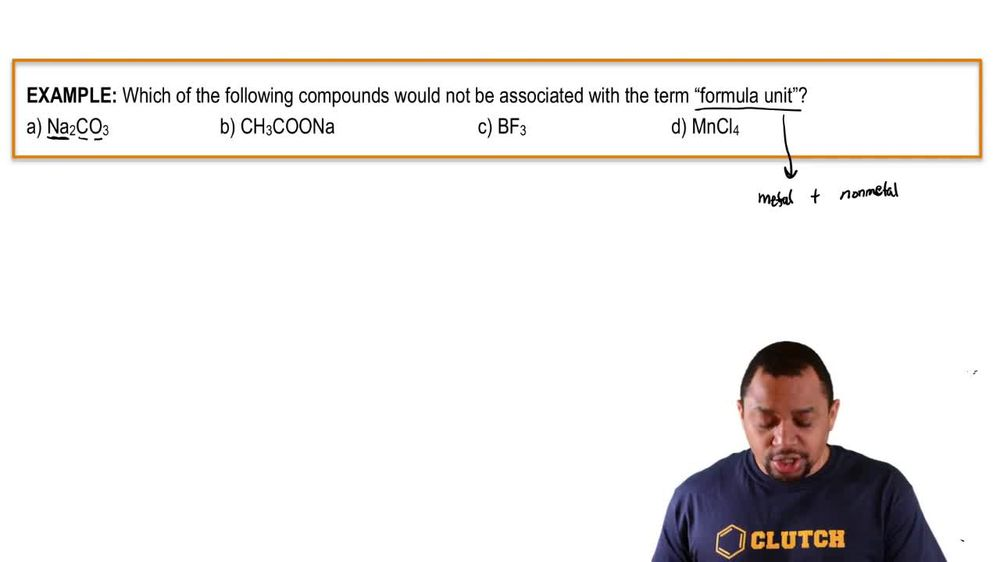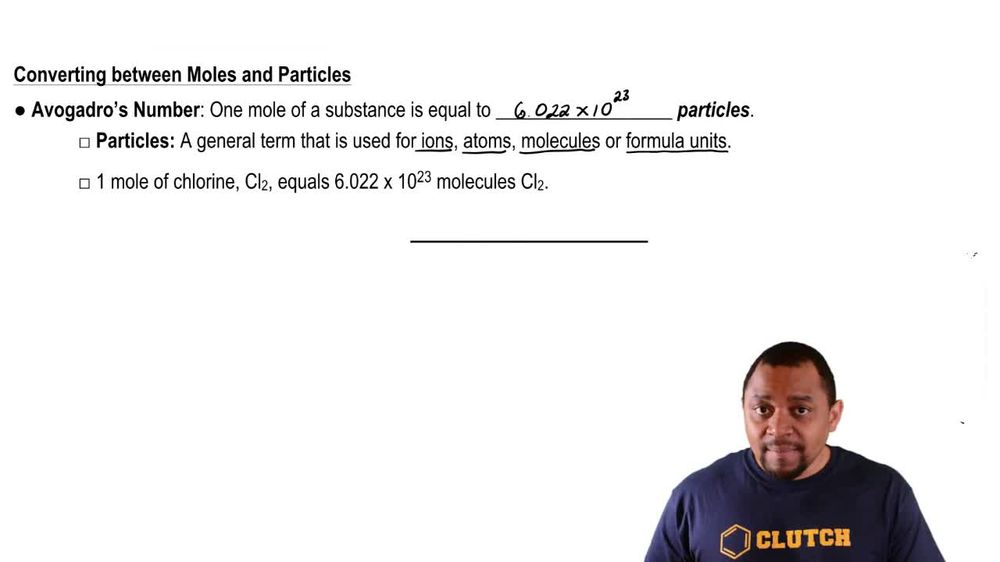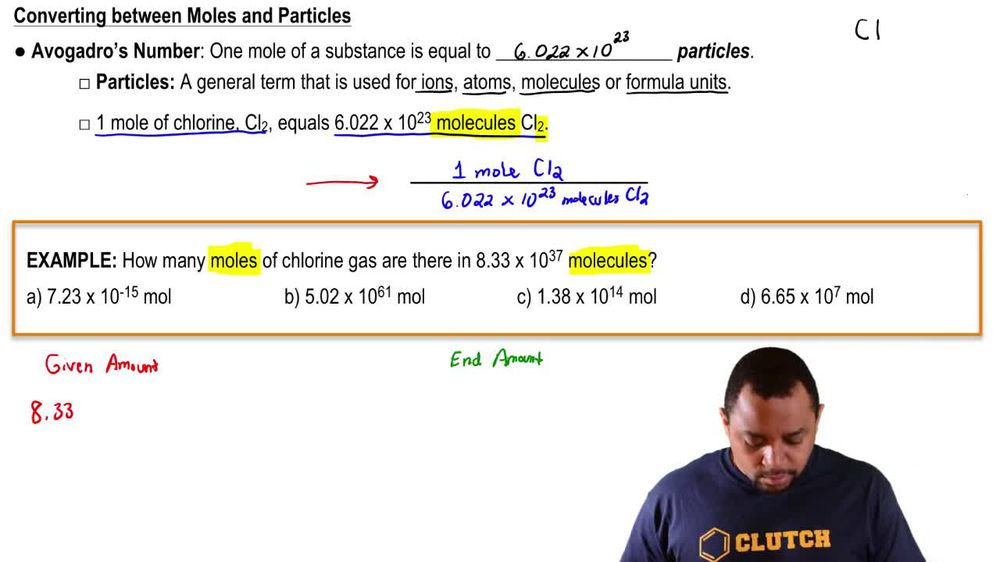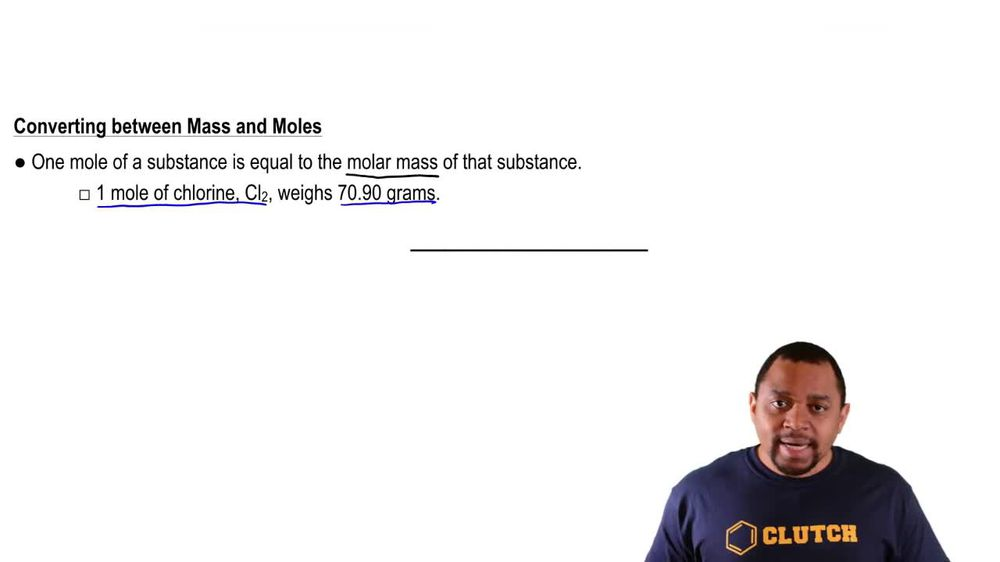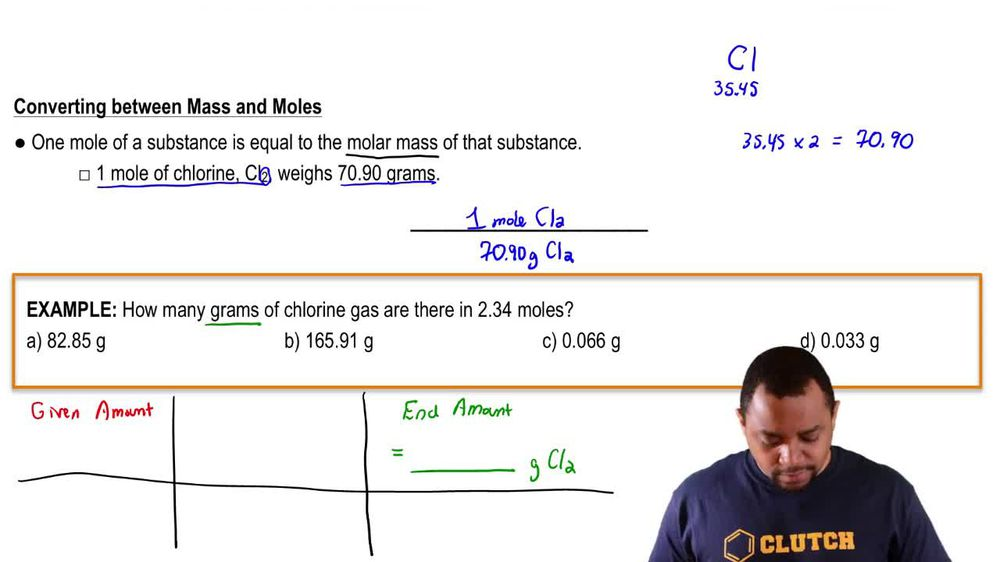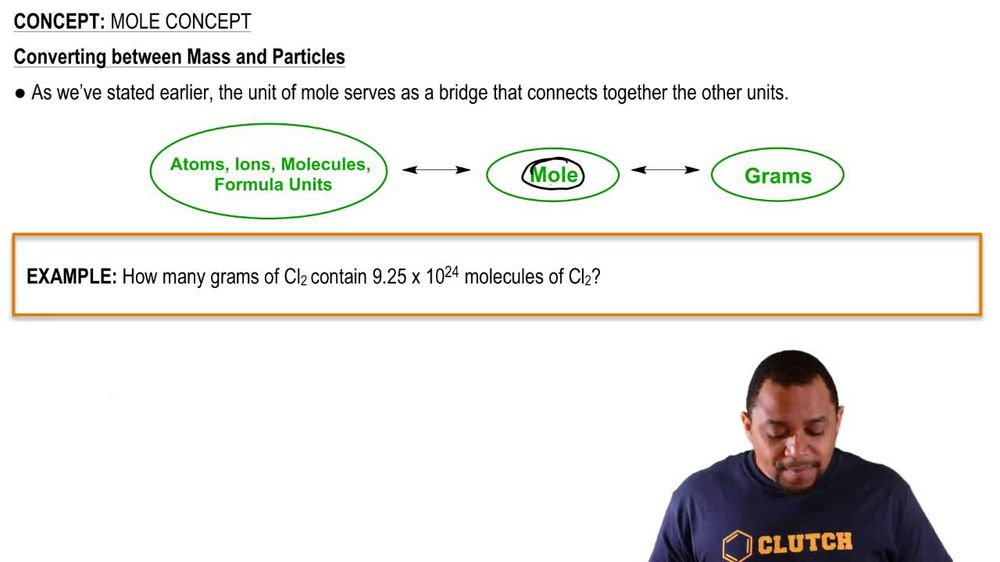Start typing, then use the up and down arrows to select an option from the list.
1. 2. Atoms & Elements2. Mole Concept
Problem

# Calculate the following quantities: (a) mass, in grams, of 0.105 mol sucrose 1C12H22O112

Relevant Solution1m
Play a video:
Hi everyone here we have a question asking us to calculate the mass and grams of 0.596 moles of galactose C six H 06. So first we need to calculate the molar mass of galactose So we have carbon and we have six of them. And if we look on the periodic table, it has a molar mass of 12.01 That equals 72.06. We have hydrogen. We have 12 of them, and it has a molar mass of 1.01 that equals 12.12. We have oxygen. We have six of them and it has a massive 16. That gives us a mass of 96. And when we add the salt together, we at 180.18 g per mole. So we're going to take our 0.596 moles Times 180.18 g Permal. And our moles are going to cancel out. And that's gonna give us 107 g. And that is our final answer. Thank you for watching. Bye.